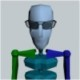# Text Along Spline Code Part IV

Continuing the code deconstruction from this post, the second pass of the algorithm is split into two parts.  The first distributes text up to the final knot in the spline.  The second part handles the case where the text extends beyond the natural spline boundaries.  This is done by using the curve’s orientation toward the end of the spline to extend the text in a straight line in that direction, as shown in this post.

The code segment for the first part of phase 2 is,

```// second pass - place text along spline
var s:Number              = 0;
var endX:Number           = __spline.getX(1);
var endY:Number           = __spline.getY(1);
var cacheRotation:Boolean = true;
var rotation:Number       = 0;

for( i=0; i<__myText.length; ++i )
{
tf = __letters[i];

if( s <= 1 )
{
var myX:Number = __spline.getX(s);
var myY:Number = __spline.getY(s);
if( s < 0.95 )
{
var dX:Number  = __spline.getX(s+0.05) - myX;
var dY:Number  = __spline.getY(s+0.05) - myY;
}
else
{
dX = endX - __spline.getX(0.94);
dY = endY - __spline.getY(0.94);
}

// normalize
var d:Number  = Math.sqrt(dX*dX + dY*dY);
var uX:Number = dX/d;
var uY:Number = dY/d;

var myWidth:Number  = tf.textWidth;
var myHeight:Number = tf.textHeight;

tf.x = myX + myHeight*uY;
tf.y = myY - myHeight*uX;
}```

The variable, s, denotes arc length along the spline.  The variables, endX and endY record the coordinates of the spline at s=1 (or the endpoints).  The Boolean variable, cacheRotation istrue if we are using a constant (precomputed) rotation value, which is the case when the text extends beyond the end of the spline.  The rotation variable denotes the rotation of the TextField for each letter distributed along  the spline.

This block of code,

```if( s <= 1 )
{
var myX:Number = __spline.getX(s);
var myY:Number = __spline.getY(s);
if( s < 0.95 )
{
var dX:Number  = __spline.getX(s+0.05) - myX;
var dY:Number  = __spline.getY(s+0.05) - myY;
}```

estimates the tangent to the curve at the current s parameter.  The cumulative text width is multiplied by the inverse of arc-length to estimate the s-parameter corresponding to the beginning of each letter, as previously described.  We could use the Singularity method that returns the derivative of the spline at the parameter value (which is the slope of the tangent), however, I wanted this code block to be usable with other packages that draw Bezier curves.  Most of those packages do not return derivative information, so it is estimated by dy/dx ~ Δy/Δx for small Δx.

The tangent of the spline at s is used to orient the TextField to align with the tangent at that point.  This is just a starting-point for aligning the text and works reasonably well except in places where the spline has extremely high curvature.  Understanding this first approximation is helpful before delving into more complex algorithms.

A unit vector in the direction of the tangent approximation is computed next and the TextField’s rotation property is set,

```var d:Number  = Math.sqrt(dX*dX + dY*dY);
var uX:Number = dX/d;
var uY:Number = dY/d;

Note the use of the atan2() function.  The x- and y-components of the unit vector are used to compute the normal vector, which is needed to position the TextField.  This will be discussed in the next part of the deconstruction.

## 5 thoughts on “Text Along Spline Code Part IV”

1.JT says:

This is slowly starting to make sense to me. Is there a difference rendering the text ‘under’ the curve rather than ‘on top?’ thanks.

2.algorithmist says:

JT – yes, there is a difference and that will be discussed in the next post.

thanks,

– jim

3.Romko Stets says:

your work on this is awesome.

could you give me a small hint? how do you draw a spline on degrafa surface?

I’ve notice there’s a container setter which requires a flash.display.Shape as an argument…

4.Romko Stets says:

Sorry for the stupid question above. I’ve handled it 🙂 thank you for this great tutorial and the Singularity lib… haven’t tried placing letter along curve yet though 🙂